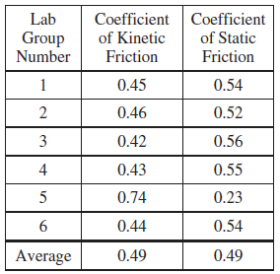# 2017 AP Physics 1物理Algebra-based真题系列之简答题免费下载

### 真题与答案下载### 真题下载请前往【纯真题】小程序### 2017 AP 物理1简答题部分免费下载

#### 部分真题预览：

2）A student wants to determine the coefficient of static friction between a long, flat wood board and a small wood block.

1. Describe an experiment for determining the coefficient of static friction between the wood board and the wood block. Assume equipment usually found in a school physics laboratory is available.
1. Draw a diagram of the experimental setup of the board and block. In your diagram, indicate each quantity that would be measured and draw or state what equipment would be used to measure each quantity.
2. Describe the overall procedure to be used, including any steps necessary to reduce experimental uncertainty. Give enough detail so that another student could replicate the experiment.
2. Derive an equation for the coefficient of static friction in terms of quantities measured in the procedure from part (a).
A physics class consisting of six lab groups wants to test the hypothesis that the coefficient of static friction between the board and the block equals the coefficient of kinetic friction between the board and the block. Each group determines the coefficients of kinetic and static friction between the board and the block. The groups’ results are shown below, with the class averages indicated in the bottom row.3. Based on these data, what conclusion should the students make about the hypothesis that the coefficients of static and kinetic friction are equal?
____The static and kinetic coefficients are equal.
____The static and kinetic coefficients are not equal.
4. A metal disk is glued to the top of the wood block. The mass of the block-disk system is twice the mass of the original block. Does the coefficient of static friction between the bottom of the block and the board increase, decrease, or remain the same when the disk is added to the block?
____Increase ____Decrease ____Remain the same

### 2017 AP 物理1模考简答题部分免费下载